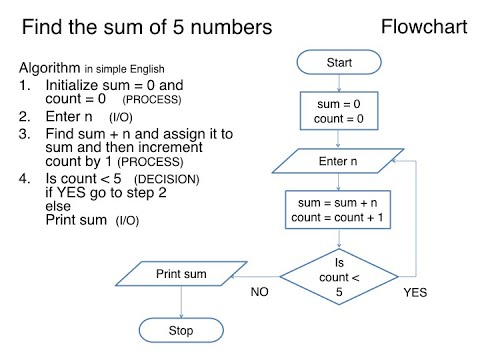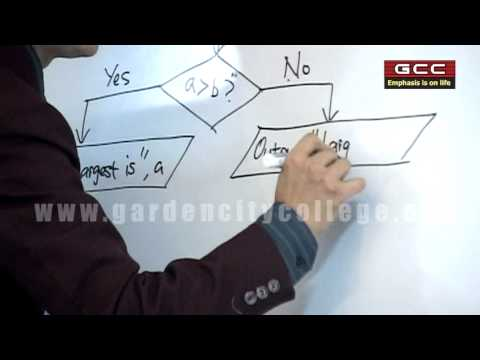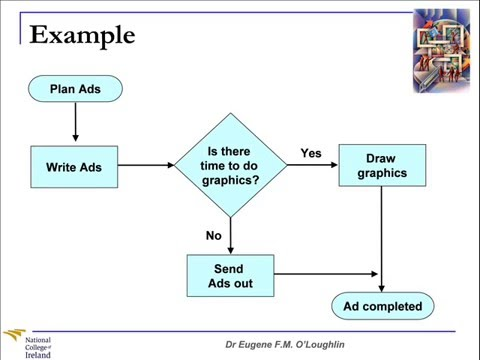## Program Logic and Procedures

Subject: Computer

#### Overview

Procedure is a mechanism of doing or performing job of task in a well suited manner or in a correct way. This note contains description on program logic and procedures.

#### Program Logic or Structure

All types of program have different module and logic. It uses three types of logic: Sequence, Selection and Iteration.

#### Sequence

Sequence is a set of computer instruction which follow one another and are executed unconditionally. In sequencing, instructions are put in a predefined order and computer executes the instructions only after the completion of previous one.

For example, a program to generate sum and product of two numbers might be as follows:

• Read the values for the variables. i.e. x and y.
• Calculate, sum = x+y
• Calculate, product= x*y
• Display the sum and product.

#### SelectionSelection is a set of computer instructions which are executed conditionally that is, they are executed on the basis of conditions that can be true or false when the program is executed.

If then
execute the statement A (no else)
If then
execute statement Aelse
execute statement B
if then
statement A
else if then
statement B
else if then
statement C¦.
else statement nth

#### Iteration (Loop)

Iteration is one in which a set of computer instructions are executed repeatedly and conditionally that is, the loop statements are driven by the loop condition. Iteration is also called looping.

Some examples of iteration (looping in programming are as follows:

#### Procedure and program

Procedure is a mechanism of doing or performing job of task in a well suited manner or in a correct way .so ,in the computer programming also ,procedure is way of defining,calling and handling various functions in an effective manner to make a program short and efficient.

Program is a set of computer instruction executed in such a manner that after the complete execution of instruction leads to completion a task or job. A program may consist of number of procedure of complete the task or solve the problem.For example,if a calculator is a program than addition is one of its function and the way of defining and calling this function is a procedure.

#### Some examples of Algorithm and Flowchart.

1. An algorithm and flowchart to generate sum of first N natural numbers.

Solution Algorithm
Step 1: Start
Step 2: Read an integer value i.e. N
Step 3: Assign, i=1.
Step 4: Calculate, Sum= Sum+ i. Increase i by 1.
Step 5: If i<=N then go to Step 4. Else go to step 6.
Step 6: Display Sum.
Step 7: End.

2. An algorithm and a flowchart to determine whether a given number is odd or even.

Solution Algorithm
Step 1: Start
Step 2: Read a number "n".
Step 3: Calculate, the remainder of n divided by 2 (i.e. n/2) that is rem=%2.
Step 4: If the rem=0 then Display "n" is even number and go to end. Else go to Step 5.
Step 5: Display "n" is odd.
Step 6: End.

3. Algorithm and flowchart to determine the largest of three given numbers.

Solution Algorithm
Step 1: Start.
Step 2: Read three numbers and store them as x, y, z.
Step 3: Compare x and y. If x is greater than y then go to step 6 else go to step 4.
Step 4: Compare y and z. If y is greater than z then print "y is largest" and go to step 8 otherwise go to step 5.
Step 5: Print "z is largest" and go to step 8.
Step 6: Compare x and z. If x is greater than z then print "x is largest" and go to step 7.
Step 7: Print "z is largest" and go to step 8.
Step 8: End

##### Things to remember
• Sequence is a set of computer instruction which follow one another and are executed unconditionally.
• Selection is a set of computer instructions which are executed conditionally that is, they are executed on the basis of conditions that can be true or false when the program is executed.
• Iteration is one in which a set of computer instructions are executed repeatedly and conditionally that is, the loop statements are driven by the loop condition.
• Procedure is a mechanism of doing or performing job of task in a well suited manner or in a correct way.
• It includes every relationship which established among the people.
• There can be more than one community in a society. Community smaller than society.
• It is a network of social relationships which cannot see or touched.
• common interests and common objectives are not necessary for society.
##### Videos for Program Logic and Procedures##### Algorithm using Flowchart and Pseudo code Level 1 Flowchart##### Concepts of Algorithm, Flow Chart & C Programming##### Problem Solving Techniques #8: Flow Charts

There are three types of logic used by QBASIC. They are Sequence, Selection and Iteration.

A set of computer instruction which follow one another and are executed unconditionally is called sequence.

Iteration is one in which a set of computer instructions are executed repeatedly and conditionally that is, the loop statements are driven by the loop condition. Iteration is also called looping.

A set of computer instruction executed in such a manner that after the complete execution of instruction leads to completion a task or job is called a program.

A mechanism of doing or performing job of task in a well suited manner or in a correct way is calledProcedure.